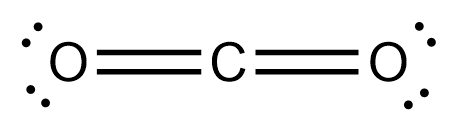# Problem: Use valence bond theory to write a hybridization and bonding scheme for CO2.Match the words in the left column to the appropriate blanks in the sentences on the right. Make certain each sentence is complete before submitting your answer.1. The Lewis structure for CO2 has a central _____ atom attached to _____ atoms through _____.2. Carbon dioxide has a _____ electron geometry.3. The carbon atom is _____ hybridized.4. Carbon dioxide has _____ π bonds and _____ δ bonds.You can only use the following words:two C(s)-O(s) ; two C(p)-O(p) ; trigonal planar ; two O(sp2)-C(sp) ; two O(sp)-C(sp2) ; carbon and oxygen ; sp2 ; a single and a double bond ; oxygen ; two single bonds ; linear ; tetrahydral ; carbon ; sp ; two double bonds ; sp3

###### FREE Expert Solution

We are asked to use valence bond theory to write a hybridization and bonding scheme for CO2.

CO2:

Carbon → Central atom → Group 4A → 4 valence electrons

O → Group 6A → 6 valence electronsElectron geometry:

2 atoms +0 lone pairs = 2 groups

AX2 Linear

Hybridization:

Subshell                   Total # of orbitals

s             _                 1 orbital

p          _ _ _              3 orbitals

d        _ _ _ _ _          5 orbitals

99% (488 ratings)###### Problem Details

Use valence bond theory to write a hybridization and bonding scheme for CO2.

Match the words in the left column to the appropriate blanks in the sentences on the right. Make certain each sentence is complete before submitting your answer.

1. The Lewis structure for CO2 has a central _____ atom attached to _____ atoms through _____.

2. Carbon dioxide has a _____ electron geometry.

3. The carbon atom is _____ hybridized.

4. Carbon dioxide has _____ π bonds and _____ δ bonds.

You can only use the following words:

two C(s)-O(s) ; two C(p)-O(p) ; trigonal planar ; two O(sp2)-C(sp) ; two O(sp)-C(sp2) ; carbon and oxygen ; sp2 ; a single and a double bond ; oxygen ; two single bonds ; linear ; tetrahydral ; carbon ; sp ; two double bonds ; sp3